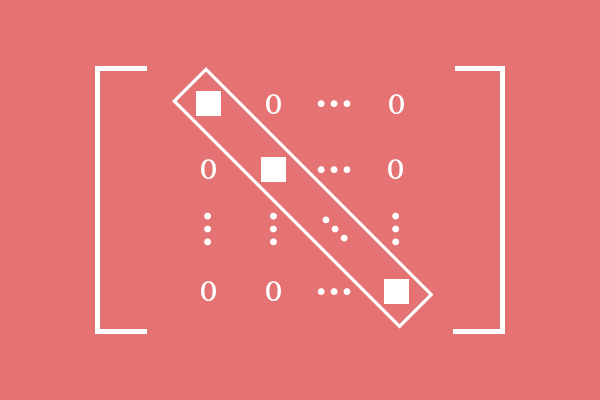# Scalar matrix

A matrix that consists of equal diagonal elements and zeros as non-diagonal entries is called a scalar matrix.

## Introduction

In a special case, each entry in the main diagonal (or leading diagonal) can be equal and the remaining non-diagonal elements can be zeros in the matrix.The following matrix is an example for this case.

$M$ $\,=\,$ $\begin{bmatrix} c & 0 & 0 & \cdots & 0\\ 0 & c & 0 & \cdots & 0\\ 0 & 0 & c & \cdots & 0\\ \vdots & \vdots & \vdots & \ddots & \vdots\\ 0 & 0 & & \cdots & c\\ \end{bmatrix}$

In English language, the word “scalar” is used to express “of a quantity”. Hence, the word “scalar” is added before the word “matrix” for expressing the equality of entries in the matrix.

Therefore, a scalar matrix is a matrix in which the diagonal elements are equal and non-zeros but the non-diagonal elements are zeros.

A scalar matrix is basically a square matrix and also a diagonal matrix due to the equality property of the entries.

$e_{11}$ $\,=\,$ $e_{22}$ $\,=\,$ $e_{33}$ $\,=\,$ $\cdots$ $\,=\,$ $e_{mm}$

When the matrix $M$ is simply written as $\begin{bmatrix} e_{ij}\\ \end{bmatrix}$, there are two conditions for calling a matrix as a scalar matrix.

1. $e_{ij} \,=\, 0$ for all $i \,\ne\, j$
2. $e_{ij} \,=\, c$ for all $i \,=\, j$ and $c \,\ne\, 0$

### Examples

The below three examples are some best examples for a scalar matrix.

$A$ $\,=\,$ $\begin{bmatrix} 6 & 0 \\ 0 & 6 \\ \end{bmatrix}$

It is a square matrix of the order $2$. In this matrix, the diagonal elements are equal and each diagonal element is $6$, and the remaining elements are zero. Hence, the matrix $A$ is called a scalar matrix.

$B$ $\,=\,$ $\begin{bmatrix} -1 & 0 & 0\\ 0 & -1 & 0\\ 0 & 0 & -1\\ \end{bmatrix}$

The matrix $B$ is a square matrix of the order $3 \times 3$. In this matrix, the diagonal entries are non-zero elements and each entry is $-1$, and the non-diagonal elements are zero. Therefore, the matrix $B$ is an example matrix for a scalar matrix.

$C$ $\,=\,$ $\begin{bmatrix} 2 & 0 & 0 & 0\\ 0 & 2 & 0 & 0\\ 0 & 0 & 2 & 0\\ 0 & 0 & 0 & 2\\ \end{bmatrix}$

The matrix $C$ is a scalar matrix of the order $4$ because the elements in the main diagonal (or principal diagonal) are non-zeros and each element in the leading diagonal is $2$ but the remaining elements are zero.

A best free mathematics education website that helps students, teachers and researchers.

###### Maths Topics

Learn each topic of the mathematics easily with understandable proofs and visual animation graphics.

###### Maths Problems

A math help place with list of solved problems with answers and worksheets on every concept for your practice.

Learn solutions

###### Subscribe us

You can get the latest updates from us by following to our official page of Math Doubts in one of your favourite social media sites.